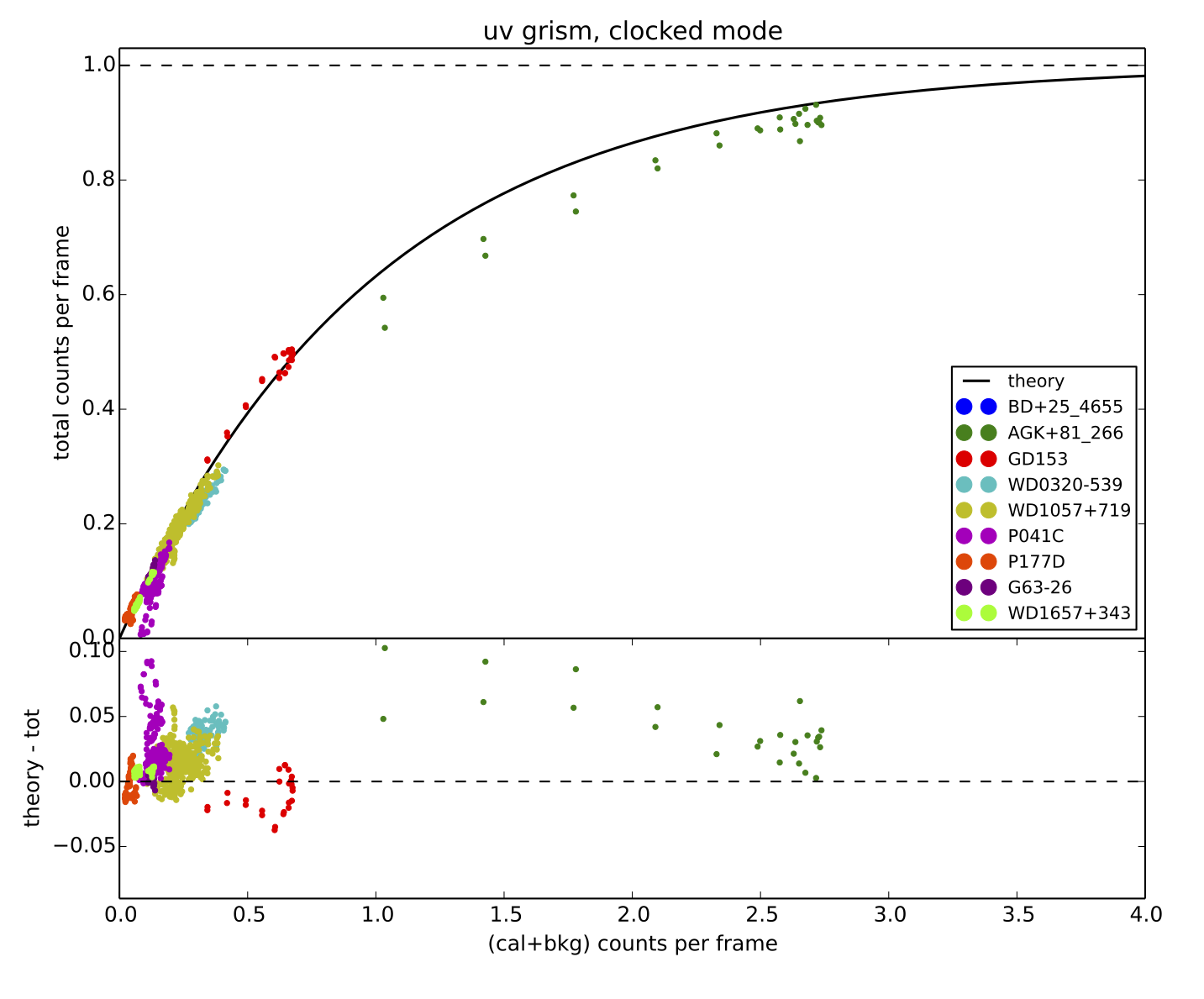# Coincidence loss in the UVOT¶

As explained in the description of the UVOT MIC detector, there is a possibility that counts incident on the detector are not counted when multiple photons are coincident on the same area on the detector.

The data are being acquired by reading out the CCD every frame time, which for the grism images is every 0.0110329 seconds. Typical exposure times range from 100-1000 seconds, which means there are a large number of frames in an exposure. Due to the finite number of frames in any exposure the statistics of incident photons, which follow a binomial distribution, can be treated for the limiting case of a Poisson distribution. The position of the incident photons can be determined to 1/8 of a physical pixel on the CCD by the fast on-board centroiding of the amplified photon splash. The coincidence loss affects measurement of photons falling in a common area on the detector, the coincidence area (short coi-area) of certain size and geometry.

## Coincidence loss theory¶

Let’s define the counts per frame by the letter $$z$$, and the count rate in counts per second by the letters $$Cr$$, and the frame time as $$t_f$$. We have then,

 $z_i = Cr\ t_f$ eq.(1)

With $$z_i$$ the incident counts per frame per coi-area. If we have many frames, with a mean rate in counts per frame $$z$$, the probability of having $$k$$ counts per frame incident on a coincidence area is therefore described by Poisson’s equation:

 $P(z_i,k) = z^k\ exp(-z_i) / k!$ eq.(2)

where $$k!$$ is the factorial of $$k$$. So there is a probability of $$exp(-z_i)$$ of no detection ($$k=0$$) in a frame. If we measure:

 $z_m = Cm/N$ eq.(3)

counts counts per frame, where $$Cm$$ is the measured count rate, and $$N$$ the number of frames, this measurement equals the probability of any detection, so we get

 $z_m = (1 - exp(-z_i))$ eq.(4)

However, the count rate in the UVOT is corrected for the detector dead-time (the time taken to read out the data from the CCD, which means only during a fraction $$a = 0.984$$ of the time, incoming photons hit the coi-area on the detector.

Now we can derive the relation between measured counts per frame and the incident photons per frame as

 $z_i = (-1/a)\ ln(1-z_m)$ eq.(5)

where $$ln$$ is the natural logarithm.

## Determination from observations¶

The relation of equation 5 can be converted into that for the count rate per second using equation 3.

For astronomical calibration sources with a known flux we can convert that flux into a prediction of the count rate by dividing by the photon energy. Once we measure that source we can relate the measured count rate to the predicted one. The following four figures show that for the four UVOT grism modes. Since one particular source only covers a limited region in count rates in the spectra, calibration sources for a range of brightness have been used.

## Figure 1: observed and predicted rates¶For the UV grism clocked mode, the observed count rate in a coi-area per frame is plotted against the incoming counts per frame. Since the predicted count rate derived from the calibration spectrum does not include the background, the background from the observed image was added after making an appropriate correction for the coincidence loss in the background. The theoretical relation from equation 5 is shown as a black line.

## The coincidence loss area¶

The maximum number of counts per frame per coi-area is one. If the coi-area is too small, the observed count rates are too low; conversely, if the coi-area is too high, the observed count rates are too high. We discuss more in

## Figures 2,3,4: observed and predicted rates for the other detector modes¶

Some of the very bright sources deviated from the overall curve. This kind of problem is not completely unexpected because the coincidence loss distorts the spectrum very significantly.

Extremely bright stars can flood the detector so that a significant part of the spectrum saturates the detector in adjacent (physical) pixels. That means that the centroiding fails, since that requires one pixel to be brighter than its neighbors. Once the centroiding fails, no count will be registered. This leads the very brightest sources to register less than the maximum count rate per frame of one. We observe that an incident count rate per frame larger than about 7 starts showing a decreased observed maximum rate.

For studying the coi-effect the spectra were split into adjacent areas extending over a length $$L_{coi}$$. A range of width $$W_{coi}$$ were chosen. For each area the average observed count rate was determined. That count rate differs from the count rate determined within the aperture of the spectrum, as it is used for calculating a correction factor for the coincidence loss only. We refer to this as the “observed coi count rate”.